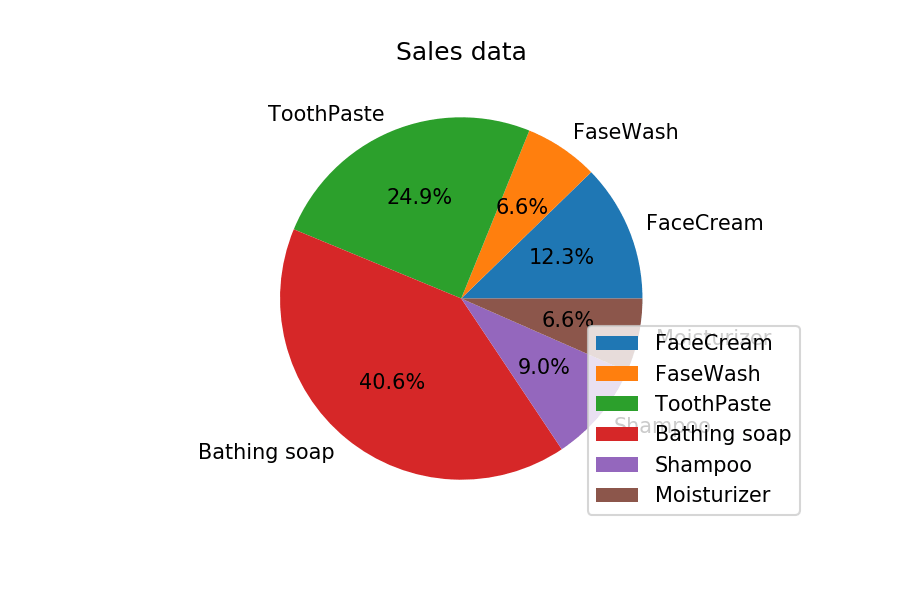Q:

# Calculate total sale data for last year for each product and show it using a Pie chart

### Calculate total sale data for last year for each product and show it using a Pie chart

Note: In Pie chart display Number of units sold per year for each product in percentage.

The Pie chart should look like this.``````import pandas as pd
import matplotlib.pyplot as plt

monthList  = df ['month_number'].tolist()

labels = ['FaceCream', 'FaseWash', 'ToothPaste', 'Bathing soap', 'Shampoo', 'Moisturizer']
salesData   = [df ['facecream'].sum(), df ['facewash'].sum(), df ['toothpaste'].sum(),
df ['bathingsoap'].sum(), df ['shampoo'].sum(), df ['moisturizer'].sum()]
plt.axis("equal")
plt.pie(salesData, labels=labels, autopct='%1.1f%%')
plt.legend(loc='lower right')
plt.title('Sales data')
plt.show()``````